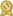Found 3779 calculus tutors – page 15 of 378.

Dunedin 34698 · \$35/hour · teaches Biology - Calculus - Elementary Math

I have successfully completed many courses at a college level including English/composition, calculus, chemistry 1 and 2, physics 1 and 2, biology, psychology, sociology, and many more.certified

Harrington Park 07640 · \$80/hour · teaches Algebra 2 - Calculus - Geometry - Prealgebra

I have extensive experience with algebra I and II, calculus, AP calculus, differential equations, linear algebra, trigonometry, and I have tutored AP statistics. In my previous career before setting out...certified

Arlington 76017 · \$50/hour · teaches Algebra 1 - Algebra 2 - Biology - Vocabulary -

Among these classes are: Calculus I-IV, Differential Equations, Numerical Methods, Geology, Geophysics, Fluid Mechanics, Reservoir Fluid Properties, etc. I have knowledge of grade school math (pre-algebra...certified

Beachwood 44122 · \$45/hour · teaches Algebra 2 - Calculus - Chemistry - Microsoft Excel

Specific assistance for Calculus AB/BC, AP Computer Science A, PAP & AP Chemistry & Physics Includes PSAT, SAT, SAT Math Level I & II Subject Tests. -“High School” students have come from Rocky River...certified

Ashburn 20148 · \$74/hour · teaches Algebra 2 - Calculus - Microsoft Excel - Geometry -

I have taught courses in Algebra, Statistics, Probability, Pre-Calculus, Calculus, Functions, Linear Algebra, and Math Modeling. Along with teaching, I have been a judge for the..certified

Woodland Hills 91367 · \$250/hour · teaches Algebra 2 - Calculus - Vocabulary - Geometry

I specialize in tutoring in Mathematics: Algebra 1, Algebra 2, Geometry, Pre-Calculus, AP Calculus AB/BC, Statistics, SAT and ACT... as well as physics, chemistry, biology. My SAT students have seen as much as 200...certified

Richboro 18954 · \$80/hour · teaches Algebra 2 - Calculus - Prealgebra - Precalculus -

Peter offers algebra, pre-calculus, SAT math, AP calculus,... Dr. Peter K., Oxford University PhD, has tutored over 150 students during the last 20 years, from 4th grade to graduate level, including students...certified

Los Alamitos 90720 · \$55/hour · teaches Biology - Calculus - Chemistry - Grammar -

Having tutored many students in mathematics, I have covered subjects including Algebra and Geometry, Pre-Calculus, Calculus, and Statistics. Bringing a positive attitude and fun to learning the subject of math my goal.certified

Spring 77379 · \$40/hour · teaches Algebra 1 - Algebra 2 - Precalculus - Trigonometry

She was not only my teacher, but also a great tutor whom I lookedupp to and always reminded myself of to try harder in math. Days after days, I got better, and I loved learning math ever since after my seventh...certified

San Jose 95110 · \$65/hour · teaches Algebra 2 - Calculus - Microsoft Excel - General

My area of specialization includes electrical engineering, electronics, maths, pre-algebra, pre calculus, calculus 1, Calculus II and Calculus III, science, algebra, and trigonometry.certified